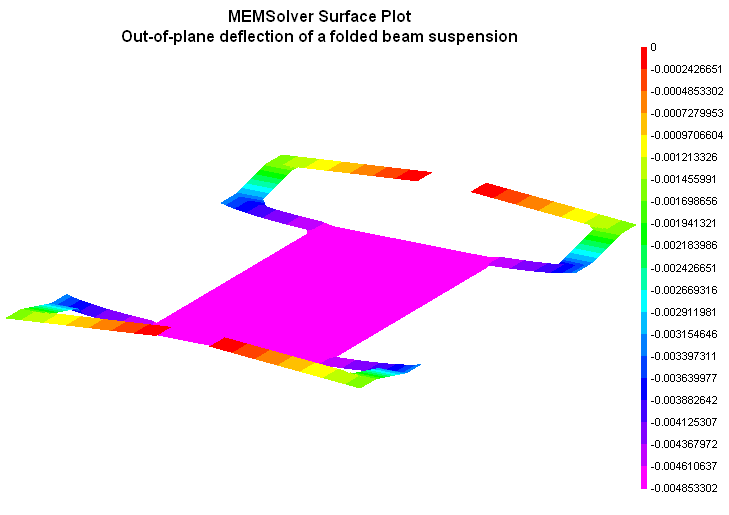FOLDBEAM,len1,len2,len3,w,t,ymod,pois,sel
Design of a folded beam suspension for planar motion

len1       length of first leg of the folded beam in µm
len2       length of second leg of the folded beam in µm
len3       length of third leg of the folded beam in µm
w           width of the beam in µm
t            thickness of the beam in µm
ymod    Young's modulus of the material of the beam in GPa
pois       Poisson's ratio
sel         number denoting the selected result.
Use 1 for spring constant in X axis and 2 for spring constant in Y axis and 3 for spring constant in Z axisNotes

The folded beam is composed of three short beam segments folded around in the shape of a 'U' and attached to the corner of the central mass. This is designed to enable a translatory motion of the suspension in the X axis or in an in-plane axis. There is however a movement in the Z axis perpendicular to the plane.

The stiffness of this beam in all the three axes directions can be found out using this design interface. The length of the three segments can be optimized to minimize the stiffness in the X axis and increase it in the other axes.

The stiffness of a single folded beam in all three axes are calculated.  If the spring constant of one beam is k1 and the second spring is k2, and if they are connected in parallel, the effective spring constant

Kparallel = k1 + k2.

If the two springs are connected in series the effective spring constant is

1/Kseries  = 1/k1 + 1/k2

In the picture four such folded beams are connected in parallel to form the suspension. If the spring constant of one beam is K and all the four beams are identical, then the effective spring constant of the suspension will be 4K.

The X-Y plot shows how the spring constants in all the three axes vary with the length of the mid-section (Len2) of a single folded beam while all other design parameters are as given in the design form. The X axis of the plot is Len2 as a percentage of Len1. Using the cross hair the value for stiffness in any of the 3 axes can be found out. It can be used to design the beam such that the stiffness for a particular axis is lesser or greater than the other axis or even the stiffness in all the axes can be made equal. This will ensure that the suspended mass will have a higher tendency to move in the required axis.

The 2D and 3D surface plots show the out-of plane deflection of the folded beam suspension.Assumptions

-The default material is Silicon with a Young's modulus of 180GPa and Poisson's ratio of 0.3.
-The beam has uniform rectangular cross section, width greater than thickness.
-Only one beam is considered in the analysis.
-In single beam analysis, the point where the mass is attached is assumed to be free to move.
-Only bending effects are considered. Axial deformations are assumed to be small compared to bending effects.